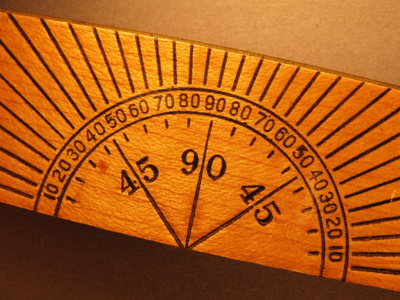A 45 degree angle is known as an acute angle.

# Level 5-6 Shapes - Angles

Shapes. They form an important part of KS3 Maths. There are many aspects to shapes, like sides, corners and area. This quiz looks at angles.

There are certain 'rules' about angles. For example, the angles in a triangle always add up to 180o. There are also different names for different types of angles - acute, right-angled, obtuse and reflex.

Can you tell an acute angle from an obtuse angle? Do you know how many degrees there are in a circle or in a square? Are you familiar with the angles of a regular pentagon or a regular hexagon? Well, this quiz will find out!

1.
What is 'angle A' in the equilateral triangle below?
50o
60o
70o
80o
The angles in a triangle add up to 180o
2.
The angle in the picture below is a(n) .......
acute angle
obtuse angle
reflex angle
right angle
Acute angles are less than 90o
3.
The angle in the picture below is a(n) .......
acute angle
obtuse angle
reflex angle
right angle
Right angles are exactly 90o
4.
What is 'angle A' in the regular pentagon below?
80o
88o
92o
108o
All angles in a regular pentagon are the same. (The length of the sides are also the same)
5.
What is 'angle A' in the diagram below?
55o
65o
75o
85o
90o + 195o + 75o = 360o
6.
The angle in the picture below is a(n) .......
acute angle
obtuse angle
reflex angle
right angle
Reflex angles are between 180o and 360o
7.
The angle in the picture below is a(n) .......
acute angle
obtuse angle
reflex angle
right angle
Obtuse angles are between 90o and 180o
8.
What is 'angle A' in the diagram below?
80o
100o
120o
140o
The angles on a straight line add up to 180o
9.
What is 'angle A' in the regular hexagon below?
80o
120o
180o
240o
Remember that all the angles in a regular hexagon are the same and all the lengths of the sides are the same
10.
What is 'angle A' in the diagram below?
90o
180o
210o
270o
The angles in a circle add up to 360o